This aim of this vignette is to introduce you to the neural gas method for scenario reduction, and to show you how to use the buildtree and checktree functions in the scenario package to construct scenario trees with predefined nodal structures. Many optimization problems need to allow for recourse in the contol, or the ability of the operator to change control decisions as uncertainty is reduced through time. Accounting for this in stochastic programming can be achieved by transforming input scenarios of the disturbance into a scenario tree. One way of doing this is by applying the neural gas method. This approach differs from other methods in that it allows the user to predefine and set the nodal structure of the tree.

## What is a scenario tree?

A scenario tree is a reduced form of an ensemble of scenarios or realizations of a process. The tree clusters those realizations into a set of branches with specified probabilities of occurrence. The tree is made up of $$S$$ scenarios, denoted $$s_i$$ ($$i = 1, 2,..., S$$). Each scenario contains $$t$$ nodes, denoted $$s_{i,t}$$ ($$t = 1, 2,..., T$$). At $$t = 1$$, all scenarios in the tree share the same node $$s_{i,1}$$. As $$t$$ increases, the tree begins to branch out. When $$t = T$$, all nodes belong to only one scenario. Each scenario has a probability $$P_i$$, and the sum of $$P_i$$ across all scenarios is $$1$$.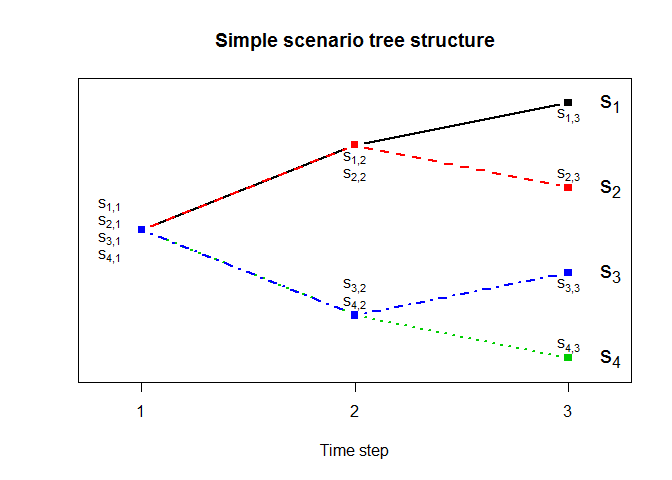The structure of a scenario tree can be represented by a scenario tree nodal partition matrix1, with the number of columns equal to the number of scenarios (4 in the above case) and the number of rows equal to the number of time steps (3 in the above case). This form of matrix is entered into the buildtree and checktree functions of the scenario package using the treeStruct parameter.

For the simple example given above, the nodes are:

##      [,1]    [,2]    [,3]    [,4]
## [1,] "S_1,1" "S_2,1" "S_3,1" "S_4,1"
## [2,] "S_1,2" "S_2,2" "S_3,2" "S_4,2"
## [3,] "S_1,3" "S_2,3" "S_3,3" "S_4,3"

Since all scenarios at $$t = 1$$ share the same node, we can state that $$S_{1,1} = S_{2,1} = S_{3,1} = S_{4,1}$$. Similarly, $$S_{1,2} = S_{2,2}$$ and $$S_{3,2} = S_{4,2}$$. Thus, the associated scenario tree nodal partition matrix can be written as:

##      [,1] [,2] [,3] [,4]
## [1,]    1    1    1    1
## [2,]    2    2    5    5
## [3,]    3    4    6    7

The easiest way to write this matrix is go column by column, raising the node integer by 1 for each node that has not already been defined. After writing a tree structure matrix, you can check if it is correct using the checktree function:

treeStruct <- rbind(c(1,1,1,1),
c(2,2,5,5),
c(3,4,6,7))
scenario::checktree(treeStruct)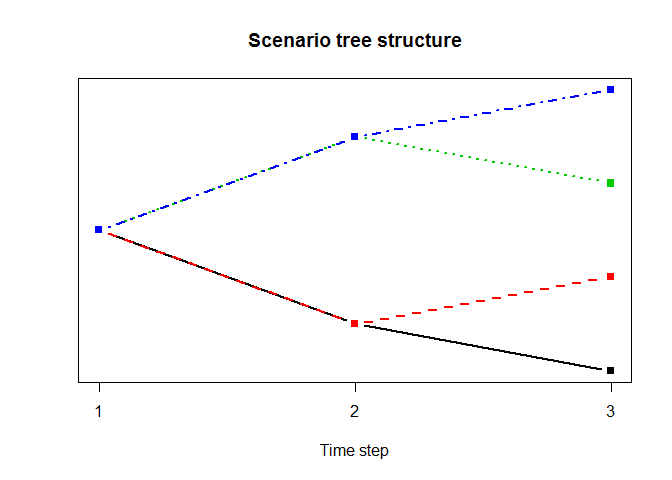If then you feel that the tree requires more or less complexity, simply alter and recheck before using the structure in the buildtree function. For example:

treeStruct <- rbind(c(1,1,1,1,1,1),
c(2,2,5,5,8,11),
c(3,4,6,7,9,12))
scenario::checktree(treeStruct)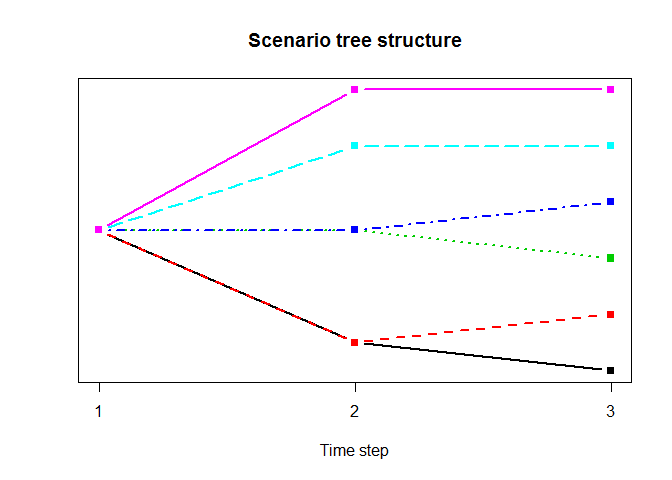## The neural gas method for scenario reduction

(Note that the following steps are set out solely to illustrate what the buildtree function does—you can start to build trees without this in-depth knowledge by simply running the function, which is described in the next section.)

From Wikipedia:

The neural gas is a simple algorithm for finding optimal data representations based on feature vectors. The algorithm was coined “neural gas” because of the dynamics of the feature vectors during the adaptation process, which distribute themselves like a gas within the data space.

The neural gas algorithm can be used to define the node values (and scenario probabilites) of a given scenario tree structure to best describe a set of input scenarios (which we will term “realizations” to distinguish from the scenarios belonging to the scenario tree). When applied to generation of a scenario tree with a predefined nodal structure, the neural gas algorithm requires three types of input. First, we need an initial set of realizations of length $$T$$, which we will term $$X$$ ($$X$$ may be an ensemble of forecasts, or simply a record of historical observed disturbances). Second, we need to define the desired scenario tree structure and then code it as a scenario tree nodal partition matrix, as described above. Third, we need some input parameters that define the resolution of the iterations taken by the algorithm. These are defaulted to recommended values in the buildtree function; the jMax parameter can be altered to trade off accurracy and computation time.

The following steps walk through the algorithm using a simple, stylized example. Here we generate the realizations artificially from a known tree, so as to illustrate the efficacy of the algorithm.

known_tree <- cbind(c(0,2,3),
c(0,2,1),
c(0,-2,-3),
c(0,-2,-1)
)
# now add some noise to the known tree...
realizations <- matrix(rep(known_tree,5), ncol=20) + matrix(rnorm(60,0,1),ncol=20)
matplot(realizations, lty=2, col = "grey", type="l",
ylab = "Disturbance", xaxt = "n", main = "Initial realizations")
axis(1, at=1:3, labels=1:3)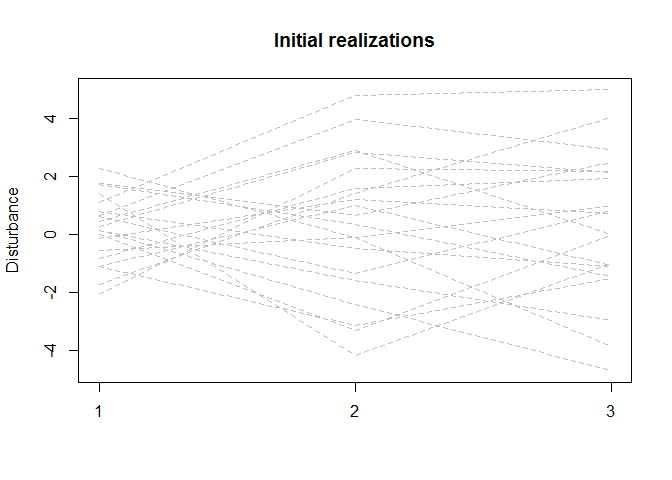#### Step 1. Initialize the scenario tree

To initialize the tree before running the iterations, the tree nodes must be given an initial values. Exactly what these values are is relatively unimportant, since the algorithm will quickly perturb the tree as it begins to fit it to the realizations. A simple way to initialize the tree is to assign each scenario the value of a randomly selected realization, $$X_k$$ ($$k = 1, 2, ..., K$$; $$K$$ is the total number of realizations—20 in the example given above). Averages are then taken to give nodes belonging to more than one scenario the same value, which ensures that the scenario tree structure is maintained. An example of an initial tree position is given below.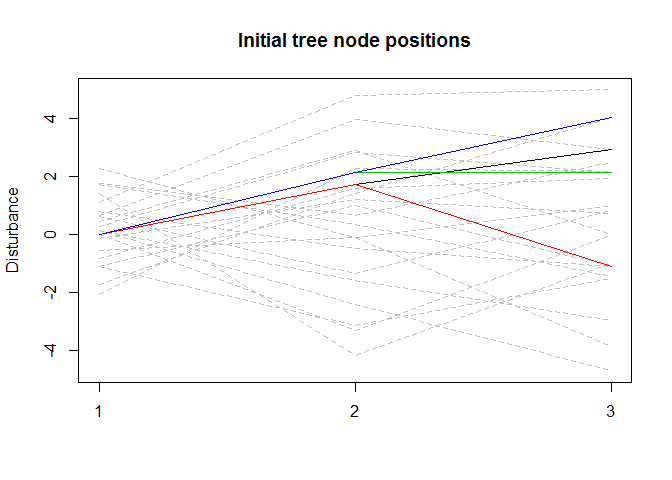The following parameters are set before beginning the iterative procedure:

$$\lambda_0$$ $$(= 10)$$

$$\lambda_f$$ $$(= 0.01)$$

$$\epsilon_0$$ $$(= 0.5)$$

$$\epsilon_f$$ $$(=0.05)$$

$$j_{max}$$ $$(=40000)$$

The brackets give the buildtree default values. Once the tree is initialized, an iteration counter $$j$$ is set to 1.

#### Step 2. Weight by Euclidean distance order

Each iteration of the neural gas procedure begins with the random selection of a realization $$X_k$$ from $$X$$. An example is given below, with the selected realization shown as a thick black dashed line.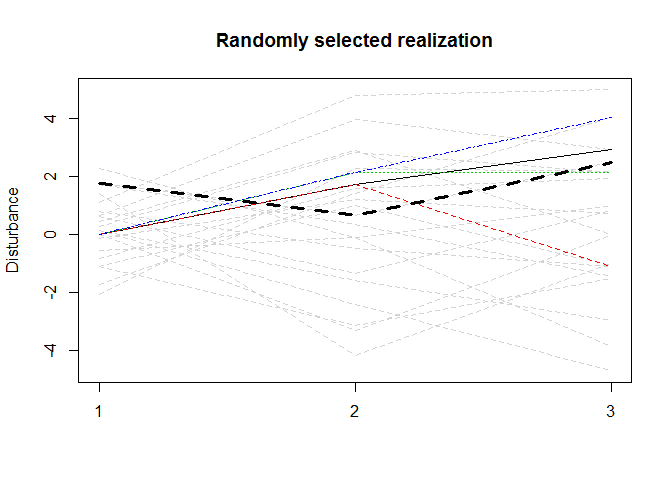The algorithm aims to determine which of the scenarios in the tree are most likely to represent the randomly chosen realization, and then move the scenarios according to those likelihoods. This is achieved by weighting each scenario according to its distance from the randomly chosen realization. To do this, we compute the Euclidean distances from the realization $$X_k$$ to each scenario ($$s_i$$, $$i = 1, 2, ..., S$$) using:

$$d_{i,k} = \sqrt{\sum_{t=1}^{T} (s_{i,t} - X_{k,t})^{2}}$$

To weight the scenarios, we use the rank of the distances. To exemplify, the Euclidean distances and scenario ranks for our simple example are:

##      Euclidean_distances Rank
## [1,]            2.122578    1
## [2,]            4.149948    4
## [3,]            2.323245    2
## [4,]            2.786486    3

The ranks are stored in vector $$R$$ for use in the following step. Those scenarios with the lowest rank (i.e., closest to the realization) will be perturbed most toward the realization. As the process iterates with new randomly chosen values, the tree will begin to spread out with each scenario moving toward the realizations it is most likely to represent.

We adjust the value of each node turn according to the following equation:

$$\Delta s_{i,t} = \epsilon(j) \cdot \sum_{i'} h(R_{i'}, \lambda(j)) \cdot (X_{k,t} - s_{i',t}) / \sum_{i'} 1$$

where $$i'$$ indexes through the scenarios that pass through node $$s_{i,t}$$ (for example, four scenarios pass through the first node of the simple tree above, so the equation sums for all four scenario ranks when applied to this node.

$$\epsilon(j) = \epsilon_0 \cdot (\epsilon_f/\epsilon_0)^{j/j_{max}}$$

$$h(R_{i'}, \lambda(j)) = e^{-R_{i'}/\lambda(j)}$$

$$\lambda(j) = \lambda_0 \cdot (\lambda_f/\lambda_0)^{j/j_{max}}$$

#### 4. Iterate

After each node is adjusted (by adding $$\Delta s_{i,t}$$ to the previous value $$s_{i,t}$$), we add 1 to $$j$$ and start the process again from step 2. The iteration is completed when $$j = j_{max}$$.

#### 5. Compute probabilites

Once the scenario tree has converged, the probabilities of each scenario are computed by assigning to each scenario a realization, based on lowest Euclidean distance. Assuming all realizations are equiprobably, the probability of any scenario is simply the proportion of total realizations that lie closer to it than any other scenario.

## Building scenario trees with buildtree

The neural gas algorithm can be executed easily using the buildtree function in scenario. The code below inputs the realizations and tree structure for the simple example case above. $$j_{max}$$ is set to 1000 for efficiency; a more representative tree can be found by increasing $$j_{max}$$.

scenario::buildtree(realizations, treeStruct, jMax = 1000)
## $tree_structure ## [,1] [,2] [,3] [,4] ## [1,] 1 1 1 1 ## [2,] 2 2 5 5 ## [3,] 3 4 6 7 ## ##$tree_values
##            [,1]        [,2]      [,3]      [,4]
## [1,]  0.2476745  0.24767448 0.2476745 0.2476745
## [2,] -1.5126028 -1.51260276 1.9245998 1.9245998
## [3,] -1.8169913  0.03721561 2.6616859 0.8105466
##
## \$branch_probabilities
##  0.35 0.15 0.35 0.15
matlines(known_tree, lwd = 2, lty = 2, col = "black")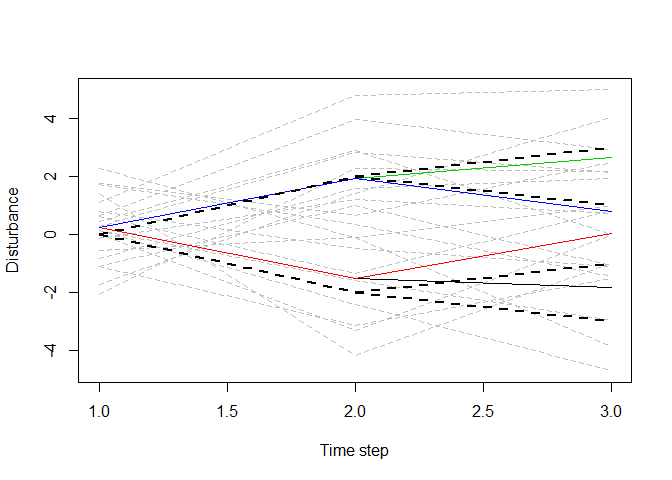Note that the function returns a list with three objects: the initial desired tree structure; the values of the final tree nodes, and the probabilities of each scenario of the tree. The figure compares the final tree against the known tree from which the realizations were generated.